### I Be Cav Home

R Lover but !a Programmer

Chuck Powell

# Writing better R functions part two – April 10, 2018

Tagged as: [ R  ggplot2  functions  dplyr  NSE  lapply  mapply  ]

In my last post I started to build two functions that took pairs of variables from a dataset and produced some nice useful `ggplot` plots from them. We started with the simplest case, plotting counts of how two variables cross-tabulate. Then we worked our way up to being able to automate the process of plotting lots of pairings of variables from the same dataframe. Today we’ll improve our functions and add a feature. For now, we’re going to continue to make use of the built in dataframe known as `mtcars`. We’re doing that to make sure that whatever we do in this post today, it works on a known start point so we can compare and contrast.

I’m going to output all the plots in a smaller size for the benefit of you the readers. I’m doing that via RMarkdown and it won’t happen automatically for you if you download and use the code. I’ll be using , fig.width=4.5, fig.height=2

## Background and catch-up

Some quick setup.

``````library(dplyr)
library(ggplot2)
theme_set(theme_bw()) # set theme to my personal preference
``````

We started with a simple task in the console. Take two of the `mtcars` variables, in this case `am` and `cyl`, and conduct a cross tabulation and then plot it.

``````### with dplyr and ggplot manually
mtcars %>%
filter(!is.na(am), !is.na(cyl))  %>%
group_by(am,cyl) %>%
count() %>%
ggplot(aes(fill=am, y=n, x=cyl)) +
geom_bar(position="dodge", stat="identity")
``````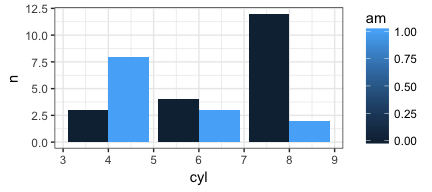By the end of the last post we had accomplished the following tasks:

1. Our function (called `PlotMe`) was a lot more robust. It checked for basic user entry errors, added a useful title and generally did what we wanted.
2. Along the way we learned the “tricks” of working with `dplyr` and `ggplot2` inside of functions.

Here’s the `PlotMe` function as we left it.

``````PlotMe <- function(dataframe,x,y){
# error checking
if (!require(ggplot2)) {
}
theme_set(theme_bw())
if (!require(dplyr)) {
}
dfname <- deparse(substitute(dataframe))
aaa <- enquo(x)
bbb <- enquo(y)
if (length(match.call()) <= 3) {
stop("Not enough arguments passed... requires a dataframe, plus two variables")
}
if (!exists(deparse(substitute(dataframe)))) {
stop("The first item in your list does not exist")
}
if (!is(dataframe, "data.frame")) {
stop("The first name you passed does not appear to be a data frame")
}
if (!deparse(substitute(x)) %in% names(dataframe)) {
stop(paste0("'", deparse(substitute(x)), "' is not the name of a variable in '",deparse(substitute(dataframe)),"'"))
}
if (!deparse(substitute(y)) %in% names(dataframe)) {
stop(paste0("'", deparse(substitute(y)), "' is not the name of a variable in '",deparse(substitute(dataframe)),"'"), call. = FALSE)
}
missing <- apply(is.na(dataframe[,c(deparse(substitute(x)),deparse(substitute(y)))]), 1, any)
if (any(missing)) {
warning(paste(sum(missing)), " row(s) not plotted because of missing data")
}
# end of error checking
dataframe %>%
filter(!is.na(!! aaa), !is.na(!! bbb))  %>%
mutate(!!quo_name(aaa) := factor(!!aaa), !!quo_name(bbb) := factor(!!bbb)) %>%
group_by(!! aaa,!! bbb) %>%
count() %>%
ggplot(aes_(fill=aaa, y=~n, x=bbb)) +
geom_bar(position="dodge", stat="identity") +
ggtitle(bquote("Crosstabs"*.(dfname)*.(aaa)~"by"*.(bbb))) +
ylab("Count") ->p
plot(p)
}
PlotMe(mtcars,am,cyl)
``````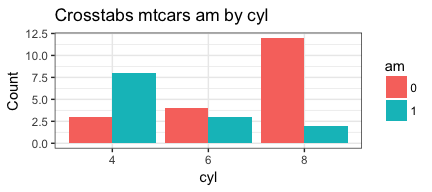## Making our function better

Our next step is to allow the user to choose which of three plot types they’d like. We have been focusing on simple side by side grouped bars that are obtained with `geom_bar(position="dodge", stat="identity")`But we can also “stack” the bars with by changing to `geom_bar(stat="identity")`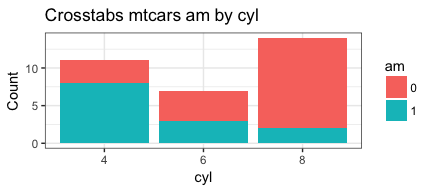Or focus on percentages of the category totals with `geom_bar(stat="identity", position="fill")`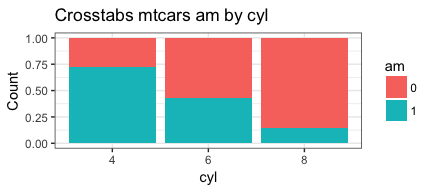The key is that our entire function is the same, except for one part of one line in the function the `geom_bar()`. The last thing we want to do is write three different functions, or replicate too much code within one function. Let’s say, for the sake of argument, we’d like to simply have the user specify whether they want type “side”, “stack” or “percent”. (We could choose any names we like but these will do for now). Let’s say, that we’d like to call this variable within our function `plottype`. We’d make `side` the default, but allow the user to override that and choose either `stack` or `percent` instead. So, at the beginning of the function we’ll add…

``````PlotMeX <- function(dataframe, x, y, plottype = "side"){
``````

Depending on their choice we’ll set the `geom_bar()` details. So it’s a kind of a `switch` hint, hint. Inside of the switch we’ll use the fact that we can pass `ggplot` list items. Each choice `"side"`, “`stack`”, or “`percent`” causes us to set two things:

1. the parameters to `geom_bar` and,
2. the Y axis label `ylab` (since in two cases it’s a count and in one it’s a percent).
``````PlotMeX <- function(dataframe, x, y, plottype = "side"){
switch(plottype,
side =  list(geom_bar(position="dodge", stat="identity"),
ylab("Count")) -> whichbar,
stack = list(geom_bar(stat="identity"),
ylab("Count")) -> whichbar,
percent = list(geom_bar(stat="identity", position="fill"),
ylab("Percent")) -> whichbar
)
``````

Notice that based upon the value going into the `switch` we are assigning the proper information to an object called `whichbar`. Later in our function we’ll insert that into our `ggplot` flow…

``````    ggplot(aes_(fill=aaa, y=~n, x=bbb)) +
whichbar +
ggtitle(bquote("Crosstabs"~.(dfname)*.(aaa)~"by"*.(bbb))) ->p
``````

Here’s our new version of the function. Temporarily named PlotMeX, and with the error checking removed just for brevity, but it’s what we want. Let’s test it against `am` by `vs` just for a variable change of pace.

``````PlotMeX <- function(dataframe, x, y, plottype = "side"){
switch(plottype,
side =  list(geom_bar(position="dodge", stat="identity"),
ylab("Count")) -> whichbar,
stack = list(geom_bar(stat="identity"),
ylab("Count")) -> whichbar,
percent = list(geom_bar(stat="identity", position="fill"),
ylab("Percent")) -> whichbar
)

aaa <- enquo(x)
bbb <- enquo(y)
dfname <- deparse(substitute(dataframe))
dataframe %>%
filter(!is.na(!! aaa), !is.na(!! bbb))  %>%
mutate(!!quo_name(aaa) := factor(!!aaa), !!quo_name(bbb) := factor(!!bbb)) %>%
group_by(!! aaa,!! bbb) %>%
count() %>%
ggplot(aes_(fill=aaa, y=~n, x=bbb)) +
whichbar +
ggtitle(bquote("Crosstabs"*.(dfname)*.(aaa)~"by"*.(bbb))) ->p
plot(p)
}
PlotMeX(mtcars,am,vs)
``````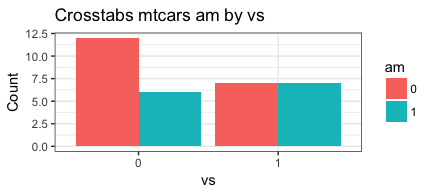``````PlotMeX(mtcars,am,vs, "stack")
``````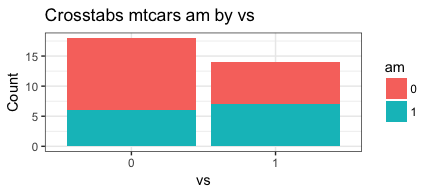``````PlotMeX(mtcars,am,vs, "percent")
``````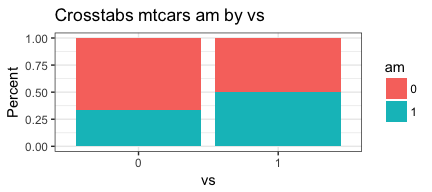Looks good!

### Automating even more

A few posts back we also recognized that if we need to work on multiple pairs of variables in the same dataframe (not uncommon in my field). It would be very tedious to keep running the `PlotMeX` function over and over again. So we developed a block of code that does something important for us. We feed it the column numbers of the variables we want to pair, accounting for which side of the plot (dependent or independent) we want them on, and the code produces two lists that we can systematically feed to our `PlotMeX` function and produce lots of plots.

There are any number of ways we could pass the columns, by quoted name, by bare name or as I have done with simple column numbers. I honestly prefer just looking up the numbers or a range of numbers. Far less typing for me and the ability to incorporate lots of variables with the colon `:` shortcut, e.g. `3:333`.

So the process would be to choose some variables for one side of the `by`, in this case let’s say `gear` and `carb` those are columns 10 & 11 so we can write `c(10:11)` on the other side `cyl` & `am` written as `c(2,9)`.

``````# Build two vectors
xwhich <- c(10:11) # let's put gear and carb in here
ywhich <- c(2,9) # let's put cyl and am in here
``````

Then we create two empty lists that we will insert the variable names into and a counter variable (not strictly necessary but I’ll actually use it in a minute). We could of course rewrite our function to accept column numbers instead of variable names right from the outset. But I’m choosey. When I work in small batches I want to be able to use names, it’s only when I have a lot of names I prefer to operate by number. It is essential to initialize the lists, and if I were ever to intend to have large lists it would be preferable to specifiy a length in advance for performance reasons. Something like ```indvars <- vector("list", length(xwhich)*length(ywhich))``` but for now I’m going to keep it simple.

``````indvars<-list() # create empty list to add to
depvars<-list() # create empty list to add to
totalcombos <- 1 # keep track of where we are
``````

Now we take `xwhich` and `ywhich` and run them through some nested loops and see if we get the four possible pairings we are expecting.

``````# loop through the vectors and build our lists
for (j in seq_along(xwhich)) {
for (k in seq_along(ywhich)) {
depvars[[totalcombos]] <- as.name(colnames(mtcars[xwhich[[j]]]))
indvars[[totalcombos]] <- as.name(colnames(mtcars[ywhich[[k]]]))
cat("iteration #", totalcombos,
" xwhich is ", xwhich[[j]], " so depvars = ", as.name(colnames(mtcars[xwhich[[j]]])),
" ywhich is ", ywhich[[k]], " so indvars = ", as.name(colnames(mtcars[ywhich[[k]]])),
"\n", sep = "")
totalcombos <- totalcombos +1
}
}
``````
``````## iteration #1 xwhich is 10 so depvars = gear ywhich is 2 so indvars = cyl
## iteration #2 xwhich is 10 so depvars = gear ywhich is 9 so indvars = am
## iteration #3 xwhich is 11 so depvars = carb ywhich is 2 so indvars = cyl
## iteration #4 xwhich is 11 so depvars = carb ywhich is 9 so indvars = am
``````

Yes that looks like what we want. It’s a bit trivial for 4 plots but imagine the effort savings if you had 5 variables on each side. Keeping track of 25 possibilities by hand is annoying. So the simplest case of our function is `PlotMeX(mtcars, am, cyl)`. We’ll feed our function and our two lists to `mapply` and let it do the tedious work for us. N.B. If you don’t wrap it with `invisible` `mapply` will actually return two copes of the plots. More on that in a bit.

``````#PlotMeX(mtcars,am,cyl)
invisible(mapply(PlotMeX, x=indvars, y=depvars, MoreArgs = list(dataframe=mtcars), SIMPLIFY = FALSE, USE.NAMES = FALSE))
``````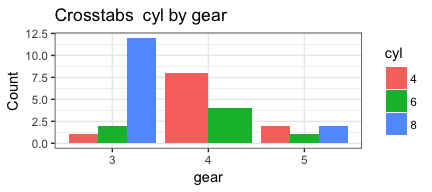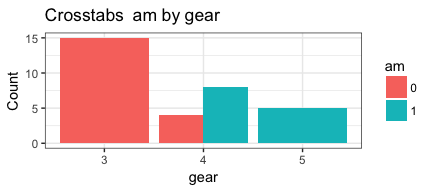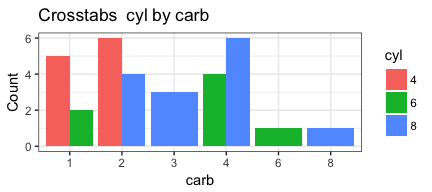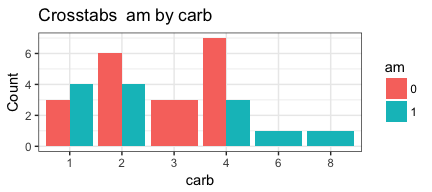Notice that `MoreArgs` is a list. We are passing it the name of the `mtcars` dataset but we can also pass it other parameters such as our `plottype` preference.

``````invisible(mapply(PlotMeX, x=indvars, y=depvars, MoreArgs = list(dataframe=mtcars, plottype = "percent"), SIMPLIFY = FALSE, USE.NAMES = FALSE))
``````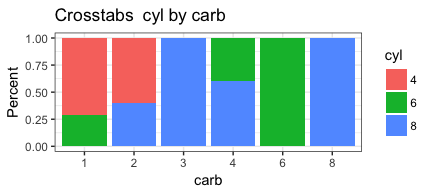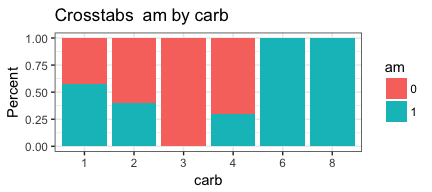Now we can turn this working code into a function so we can reuse it. To do that we’ll have the user specify the `dataframe`, `xwhich` and `ywhich` as parameters to the function. Then inside the function we’ll do the work and return the user the two lists that can be used to “feed” `mapply`. I’m personally a fan of providing some feedback in the console to inform the user of what’s going on, even though the actual output product of the function is just a list with two sublists nested inside. On the other hand we’ll return the list “invisibly” since we don’t really need to print out the list structure.

``````CrossXYs <- function(dataframe, xwhich, ywhich){
# Build two vectors
indvars<-list() # create empty list to add to
depvars<-list() # create empty list to add to
totalcombos <- 1 # keep track of where we are
message("Creating the variable pairings...")
for (j in seq_along(xwhich)) {
for (k in seq_along(ywhich)) {
depvars[[totalcombos]] <- as.name(colnames(dataframe[xwhich[[j]]]))
indvars[[totalcombos]] <- as.name(colnames(dataframe[ywhich[[k]]]))
cat("Pairing #", totalcombos, " ", as.name(colnames(dataframe[xwhich[[j]]])),
" with ", as.name(colnames(dataframe[ywhich[[k]]])), "\n", sep = "")
totalcombos <- totalcombos +1
}
}
return(invisible(list(depvars=depvars,indvars=indvars)))
}
CrossXYs(mtcars,c(10:11),c(2,9))
``````
``````## Creating the variable pairings...

## Pairing #1 gear with cyl
## Pairing #2 gear with am
## Pairing #3 carb with cyl
## Pairing #4 carb with am
``````

This code produces the two lists with the column names varying in the way we want them. So the next step it to put them somewhere (we’ll call that `bothlists`)

``````bothlists <- CrossXYs(mtcars,c(10:11),c(2,9))
``````
``````## Creating the variable pairings...

## Pairing #1 gear with cyl
## Pairing #2 gear with am
## Pairing #3 carb with cyl
## Pairing #4 carb with am
``````

Then we can use them as part of our `mapply` call by addressing them as `bothlists\$indvars` & `bothlists\$depvars`, otherwise our command remains the same.

``````invisible(mapply(PlotMeX, x=bothlists\$indvars, y=bothlists\$depvars, MoreArgs = list(dataframe=mtcars, plottype = "stack"), SIMPLIFY = FALSE, USE.NAMES = FALSE))
``````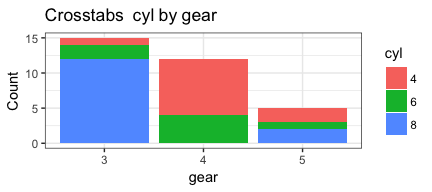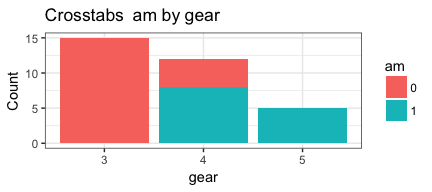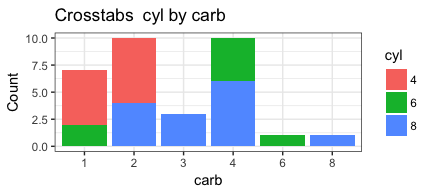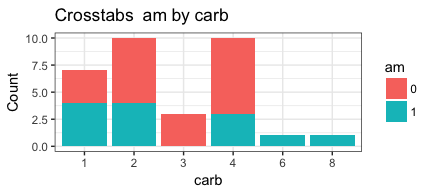Appears to be working as we want it to. Which means it’s a good time to test it on some other data and see if anything breaks. Let’s try the esoph dataset… First we’ll call `PlotMeX` with `alcgp` by `tobgp`. Then we’ll make `CrossXYs` give us lists for `agegp` by `alcgp` & `tobgp`. That should reassure us that all is well.

``````str(esoph)
``````
``````## 'data.frame':    88 obs. of  5 variables:
##  \$ agegp    : Ord.factor w/ 6 levels "25-34"<"35-44"<..: 1 1 1 1 1 1 1 1 1 1 ...
##  \$ alcgp    : Ord.factor w/ 4 levels "0-39g/day"<"40-79"<..: 1 1 1 1 2 2 2 2 3 3 ...
##  \$ tobgp    : Ord.factor w/ 4 levels "0-9g/day"<"10-19"<..: 1 2 3 4 1 2 3 4 1 2 ...
##  \$ ncases   : num  0 0 0 0 0 0 0 0 0 0 ...
##  \$ ncontrols: num  40 10 6 5 27 7 4 7 2 1 ...
``````
``````PlotMeX(esoph,alcgp,tobgp)
``````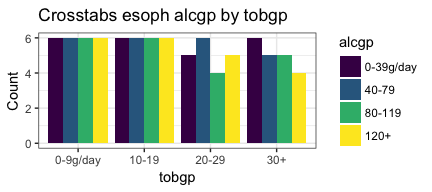``````bothlists <- CrossXYs(esoph,1,c(2:3))
``````
``````## Creating the variable pairings...

## Pairing #1 agegp with alcgp
## Pairing #2 agegp with tobgp
``````
``````invisible(mapply(PlotMeX, x=bothlists\$indvars, y=bothlists\$depvars, MoreArgs = list(dataframe=esoph, plottype = "percent"), SIMPLIFY = FALSE, USE.NAMES = FALSE))
``````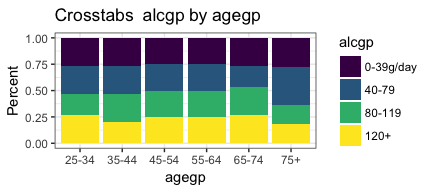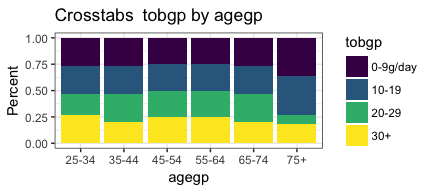Very nice! We seem to have accomplished what we set out to do.

## All done (not yet!)

This has become a very long post so I’m going to end here. But the astute among you have probably detected we’re still being inefficient. Be nice if we could integrate these two functions a little better so we didn’t have to think of it as a two step process. Great idea for a next post on a future day.

I hope you’ve found this useful. I am always open to comments, corrections and suggestions.

Chuck (ibecav at gmail dot com)